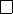5Eigenvalues and eigenvectors

IA Vectors and Matrices5.2 Linearly independent eigenvectors
Theorem.
Suppose
n×n
matrix
A
has distinct eigenvalues
λ
1
, λ
2
, ··· , λ
n
. Then
the corresponding eigenvectors x
1
, x
2
, ··· , x
n
are linearly independent.
Proof.
x
1
, x
2
, ··· , x
n
are linearly dependent.
Then we can find non-zero constants d
i
for i = 1, 2, ··· , r, such that
d
1
x
1
+ d
2
x
2
+ ··· + d
r
x
r
= 0.
Suppose that this is the shortest non-trivial linear combination that gives
0
(we
may need to re-order x
i
).
Now apply (A λ
1
I) to the whole equation to obtain
d
1
(λ
1
λ
1
)x
1
+ d
2
(λ
2
λ
1
)x
2
+ ··· + d
r
(λ
r
λ
1
)x
r
= 0.
We know that the first term is
0
, while the others are not (since we assumed
λ
i
6= λ
j
for i 6= j). So
d
2
(λ
2
λ
1
)x
2
+ ··· + d
r
(λ
r
λ
1
)x
r
= 0,
and we have found a shorter linear combination that gives
0
Example.
(i) A =
0 1
1 0
. Then p
A
(λ) = λ
2
+ 1 = 0. So λ
1
= i and λ
2
= i.
To solve (A λ
1
I)x = 0, we obtain
i 1
1 i
x
1
x
2
= 0.
So we obtain
x
1
x
2
=
1
i
to be an eigenvector. Clearly any scalar multiple of
1
i
is also a solution,
but still in the same eigenspace E
i
= span
1
i
Solving (A λ
2
I)x = 0 gives
x
1
x
2
=
1
i
.
So E
i
= span
1
i
.
Note that
M
(
±i
) =
m
(
±i
) = 1, so
±i
= 0. Also note that the two
eigenvectors are linearly independent and form a basis of C
2
.
(ii) Consider
A =
2 2 3
2 1 6
1 2 0
Then
det
(
A λI
) = 0 gives 45 + 21
λ λ
2
λ
3
. So
λ
1
= 5
, λ
2
=
λ
3
=
3.
The eigenvector with eigenvalue 5 is
x =
1
2
1
We can find that the eigenvectors with eigenvalue 3 are
x =
2x
2
+ 3x
3
x
2
x
3
for any
x
2
, x
3
. This gives two linearly independent eigenvectors, say
2
1
0
,
3
0
1
.
So
M
(5) =
m
(5) = 1 and
M
(
3) =
m
(
3) = 2, and there is no defect for
both of them. Note that these three eigenvectors form a basis of C
3
.
(iii) Let
A =
3 1 1
1 3 1
2 2 0
Then 0 =
p
A
(
λ
) =
(
λ
+ 2)
4
. So
λ
=
2
,
2
,
2. To find the eigenvectors,
we have
(A + 2I)x =
1 1 1
1 1 1
2 2 2
x
1
x
2
x
3
= 0
The general solution is thus
x
1
+
x
2
x
3
= 0, and the general solution is
thus x =
x
1
x
2
x
1
+ x
2
. The eigenspace E
2
= span
1
0
1
,
0
1
1
.
Hence
M
(
2) = 3 and
m
(
2) = 2. Thus the defect
2
= 1. So the
eigenvectors do not form a basis of C
3
.
(iv)
Consider the reflection
R
in the plane with normal
n
. Clearly
Rn
=
n
.
The eigenvalue is
1 and the eigenvector is
n
. Then
E
1
=
span{n}
. So
M(1) = m(1) = 1.
If
p
is any vector in the plane,
Rp
=
p
. So this has an eigenvalue of 1 and
eigenvectors being any vector in the plane. So M(1) = m(1) = 2.
So the eigenvectors form a basis of R
3
.
(v)
Consider a rotation
R
by
θ
n
. Since
Rn
=
n
, we have an eigenvalue
of 1 and eigenspace E
1
= span{n}.
We know that there are no other real eigenvalues since rotation changes
the direction of any other vector. The other eigenvalues turn out to be
e
±
. If
θ 6
= 0, there are 3 distinct eigenvalues and the eigenvectors form a
basis of C
3
.
(vi) Consider a shear
A =
1 µ
0 1
The characteristic equation is (1
λ
)
2
= 0 and
λ
= 1. The eigenvectors
corresponding to
λ
= 1 is
x
=
1
0
. We have
M
(1) = 2 and
m
(1) = 1. So
1
= 1.
If
n × n
matrix
A
has
n
distinct eigenvalues, and hence has
n
linearly
independent eigenvectors
v
1
, v
2
, ···v
n
, then with respect to this eigenvector
basis, A is diagonal.
In this basis,
v
1
= (1
,
0
, ··· ,
0) etc. We know that
Av
i
=
λ
i
v
i
(no summation).
So the image of the
i
th basis vector is
λ
i
times the
i
th basis. Since the columns
of A are simply the images of the basis,
λ
1
0 ··· 0
0 λ
2
··· 0
.
.
.
.
.
.
.
.
.
.
.
.
0 0 ··· λ
n
The fact that
A
can be diagonalized by changing the basis is an important
observation. We will now look at how we can change bases and see how we can
make use of this.